# Clock hands

The hands-on clock shows the time 12 hours and 2 minutes. Calculate the size of an acute angle between clock hands three hours later.

x =  79 °

### Step-by-step explanation: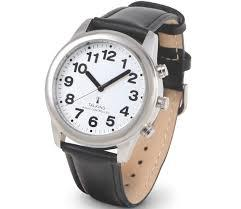Did you find an error or inaccuracy? Feel free to write us. Thank you!Tips to related online calculators
Need help to calculate sum, simplify or multiply fractions? Try our fraction calculator.
Do you have a linear equation or system of equations and looking for its solution? Or do you have a quadratic equation?
Do you want to convert time units like minutes to seconds?

## Related math problems and questions:

• HandsThe clock shows 12 hours. After how many minutes will angle between the hour and minute hand 90°? Consider the continuous movement of both hands hours.
• Clock Tower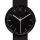What angle is betwenn hands on Clock Tower when show 17 hours and 35 minutes?
• Clock handsCalculate the internal angles of a triangle whose vertices lie on the clock's 2, 6 and 11 hours.
• Little hand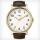What angle shifted little hand on the clock after one hour and 38 minutes?
• What time is it?What time is it, when there is four times less time left until midnight than the time that has elapsed since noon? What time is it, when one-fifth of the hours that have passed since midnight is equal to one-third of the hours that are missing by 12 o'clo
• Free timeAnita had 2 1/4 hours of free time in the afternoon. She spent 2/3 of her free time playing sports and watched TV for 30 minutes. How much time did she have left to read the book? Express the result as fractions of hours and numbers in minutes.
• ClockHow many times a day hands on a clock overlap?
• Speed of clockIn how many minutes will the hour hand pass a straight angle and a right angle?
• The big clock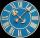The big clock hands stopped at a random moment. What is the probability that: a) a small hand showed the time between 1:00 and 3:00? b) the big hand was in the same area as a small hand in the role of a)? c) did the hours just show the time between 21:00
• LunchLunch is given to seniors from 12:15 to 12:40 during the Coronavirus pandemic. What angle will the minute hand of clock describe during this time?
• Clock mathematicsIf it is now 7:38 pm, what time will it be in 30,033,996,480 minutes from now?
• SimilarityAre two right triangles similar to each other if the first one has an acute angle 70°, and the second one has an acute angle 20°?
• Acute triangle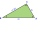In the acute triangle KLM, V is the intersection of its heights and X is the heel of height to the side KL. The axis of the angle XVL is parallel to the side LM and the angle MKL is 70°. What size are the KLM and KML angles?
• Garden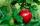Father dig up the garden in 9 hours. Son in 13 hours. How many hours take dig up the garden together?
• Tram stop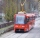The blue tram stops every 12 minutes, the red one 8 minutes. At 8 o'clock they left the stop together. How many times do they meet at a stop before 11 am?
• Grandmother's clocks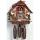Grandmother's clock is late every hour by half a minute. Grandmother set the clock exactly at 8.00 am. How many hours will show after 24 hours?
• Truck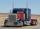Truck went for 4 hours and 30 minutes. If truck increase speed by 11 km/h, went same distance in 2 hours. Calculate this distance.